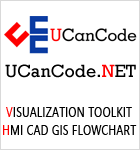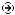YOU CAN CODE!With The Case Of UCanCode.net  Release The Power OF  Visual C++ ! Home |  Products | Purchase |  Support | DownloadsDownload EvaluationPricing & Purchase?E-XD++Visual C++/ MFC ProductsOverviewElectronic Form SolutionVisualization & HMI SolutionPower system HMI SolutionBar code labeling SolutionWorkflow SolutionCoal industry HMI SolutionInstrumentation Gauge SolutionReport Printing SolutionGraphical modeling SolutionGIS mapping solutionVisio graphics solutionIndustrial monitoring SolutionFlowchart and diagramming SolutionOrganization Diagram SolutionGraphic editor Source CodeUML drawing editor Source CodeMap Diagramming SolutionArchitectural Graphic Drawing SolutionRequest EvaluationActiveX COM ProductsOverviewTechnical Support# Get Ready to Unleash the Power of UCanCode .NET

UCanCode Software focuses on general application software development. We provide complete solution for developers. No matter you want to develop a simple database workflow application, or an large flow/diagram based system, our product will provide a complete solution for you. Our product had been used by hundreds of top companies around the world!

"100% source code provided! Free you from not daring to use components because of unable to master the key technology of components!"

 Draw Curve Line with VC++/MFC visualization Control## Introduction

• Derived from ``` CWnd```, easy to use
• Fits width and height of drawing rect
• Zooming in and out
• Mouse coordinates tracing
• Single/multiple selected by clicking mouse button
• Cross-line tracing mouse
• Add or delete a point of a curve by clicking mouse button
• Edit vertical value of a point of a curve by moving mouse with button down
• Easy to move, shift or mirror one curve
• Notify control�s owner when curve changing
• Owner determines whether to modify curve data by return values of message handle functions
• Unicode support
• Compiles under Windows 2000, VC++ 6.0 with warning level 4

## Background

Recently, I wanted to visualize data in one of my applications. I had searched from Internet and downloaded some curve controls. Most of them are beautiful and work well, but none works as I want it to be. After reading source code of some controls, I decided to develop one curve control which would meet my application�s requirements.

## Classes

#### CCurve

`CCurve` class, derived from ``` CObject``` is used to store data and curve properties. It does not perform data adding or deleting.

• ``` void ShowCurve(BOOL bShow = TRUE);```
• ```BOOL IsVisible();```

Sets/gets visualization.

• ``` void Select(BOOL bSelect = TRUE);```
• ```BOOL IsSelected```

Sets/gets selected.

• ```BOOL SetCurveName(CString& strName);```
• ``` CString GetCurveName();```

Sets/gets curve name.

• ``` void SetCurveColor(COLORREF color);```
• ``` COLORREF GetCurveColor();```

Sets/gets curve line color.

• ``` void SetCurveStyle(int iStyle);```
• ``` int GetCurveStyle();```

Sets/gets curve line style.

• ``` void SetCurveWidth(int nWidth);```
• ``` int GetCurveWidth();```

Sets/gets curve line width.

• ``` float Distance(const CPoint& pt1, const CPoint& pt2);```

Gets distance between two points.

• ```BOOL IsPointNearCurve(const CPoint& point, int& iIndex);```

Whether the point is near the curve.

#### CCurveCtrl

• ```BOOL Create(const RECT& rect, CWnd* parent, UINT nID,DWORD dwStyle = WS_CHILD | WS_BORDER | WS_TABSTOP | WS_VISIBLE);```

Creates control, where ``` rect``` is the dimensions. Refer to MSDN.

• ``` int AddCurve(const CString& strName, COLORREF color = RGB(0, 0, 0), int iStyle = PS_SOLID, int nWidth = 1);```

• ```BOOL AddData(const CString& strName, float fHori, float fVert);```
• ```BOOL AddData(CCurve* pCurve, float fHori, float fVert);```

Adds data to a curve by index or object pointer.

• ```BOOL AddCurveData(const CString& strName, const CArray< float, float >& ArrHori, const CArray< float, float >& ArrVert);```

Adds one curve and data at the same time.

• ``` CCurve* GetCurve(int iIndex);```
• ``` CCurve* GetCurve(const CString& strName);```

Gets a curve object�s pointer by its index in ``` CCurveCtrl``` or its name.

• ``` int GetIndex(const CCurve* pCurve);```

Gets a curves index in ``` CCurveCtrl``` by pointer.

• ```BOOL Remove(int index);```
• ```BOOL Remove(const CString& strName);```
• ``` void RemoveAll();```

Removes a curve or all of the curves in ``` CCurveCtrl```.

• ``` int GetCurveCount();```

Gets total count of curves.

• ``` int GetSelectedCount();```

Gets count of selected curves.

• ``` void Move(BOOL bLeft);```
• ``` void MovePage(BOOL bLeft);```

Moves all curves and coordinate scales left or right (will not modify data).

• ``` void CurveLeft(CCurve* pCurve, float fLen); void CurveRight(CCurve* pCurve, float fLen);```

Shifts a curve left or right (will modify data of the curve).

• ``` void MirrorHori(CCurve* pCurve, float fMid);```

Mirrors a curve in horizontal.

• ``` void MirrorVert(CCurve* pCurve, float fMid);```

Mirrors a curve in vertical.

• ``` void Restore();```

Shows all points of all curves.

• ```BOOL Zoom(BOOL bIn);```

Zooms in (`bIn`: ``` TRUE```) or zooms out (`bIn`: ``` FALSE```).

• ``` void SetHoriLabel(CString& str);```
• ``` CString GetHoriLabel();```

Sets/gets horizontal label.

• ``` void SetVertLabel(CString& str);```
• ``` CString GetVertLabel();```

Sets/gets vertical label.

• ``` void ShowCross(BOOL bShow);```
• ```BOOL IsShowCross();```

Whether to show cross lines.

• ``` void SetMargin(const CRect& rect);```
• ```CRect GetMargin();```

Sets/gets margin left to show title, etc.

• ``` void EnableEdit(BOOL bEdit);```
• ```BOOL CanEditCurve();```

Whether can edit curve data by using mouse.

• ``` void SetGridLineStyle(int iStyle);```
• ``` int GetGridLineStyle();```

Sets/gets style of grid line in background.

Following notify messages will be sent to controls owner window (parent or not) if curve selected/ deselected or data modified by mouse operation:

• ``` CVN_MVALUE_ADD``` - a point added to curve.
• ``` CVN_MVALUE_CHANG``` - Y value of a point changing.
• ``` CVN_MVALUE_DELETE``` - a point deleted.
• ``` CVN_CURVE_SELECTED``` - a curve is selected.
• ``` CVN_CURVE_CANCELSELECT``` - a curve is deselected.
• ``` CVN_CURVE_SELECTNONE``` - deselected all curves.

#### StructCollapseCopy Code
```typedef struct tagNM_CURVECTRL {
NMHDR  hdr;      // curve object pointer
void*  pCurve;   // curve object pointer
int    iIndex;
float  fHori;    // horizontal value where cursor is
float  fVert;    // vertical value where cursor is
} NM_CURVECTRL;```

Note the `iIndex` member: it is the index of the point in the curve if the message sent to owner is ``` CVN_MVALUE_ADD```, ``` CVN_MVALUE_CHANGE``` or ``` CVN_MVALUE_DELETE```; it is the index of the curve in ``` CCurveCtrl``` if the message sent to owner is ``` CVN_CURVE_SELECTED```, ``` CVN_CURVE_CANCELSELECT``` or ``` CVN_CURVE_SELECTNONE```.

#### Usage

Following steps demonstrate how to use ``` CCurveCtrl``` in a dialog-based application:

2. Add a ``` CCurveCtrl``` pointer member to your ``` CDialog``` class:CollapseCopy Code
`CCurveCtrl*  m_pCurveCtrl;`

And also add ``` include``` statement:CollapseCopy Code
`#include �CurveCtrl.h�`
3. Set ``` m_pCurveCtrl``` as ``` NULL``` in your dialog construction functions:CollapseCopy Code
`m_pCurveCtrl = NULL;`CollapseCopy Code
`#define ID_CURVE_CONTROL   9999`

In your ``` CDialog::OnInitDialog()```, add statements as following:CollapseCopy Code
```if (NULL == m_pCurveCtrl)
{
m_pCurveCtrl = new CCurveCtrl;
m_pCurveCtrl->Create(CRect(10, 10, 200, 200),
this,
ID_CURVE_CONTROL);    // resorce ID
m_pCurveCtrl->SetMargin(CRect(70, 50, 50, 50));
}```
4. Add one curve and data as following:CollapseCopy Code
```CString  strName(TestOne);

for (float f = 0.0f; f < 6.28f; f += 0.1f)
{
(m_nCurveCount) * f,
100.f * float(sin(f)));
}
m_pCurveCtrl->Invalidate();```

If you want to add/delete or edit points with mouse operation, you must call:CollapseCopy Code
`m_pCurveCtrl->EnableEdit(TRUE);`

Controls owner will receive notify message, and if the return values of the messages is not 0 (>0 or <0), the edit or selection /deselection attempts will be rejected.

For example, to handle the message of ``` CVN_MVALUE_CHANG```, add a handler in your dialog source file:CollapseCopy Code
```BEGIN_MESSAGE_MAP(CTestCurveDlg, CDialog)
... ...
ON_NOTIFY(CVN_MVALUE_CHANG, ID_CURVE_CONTROL, OnCurveMouseModify)
END_MESSAGE_MAP()

// message handle function
void CTestCurveDlg::OnCurveMouseModify(NMHDR *pNotifyStruct, LRESULT* pResult)
{
NM_CURVECTRL* pItem = (NM_CURVECTRL*)pNotifyStruct;
CCurve* pCurve = (CCurve*)pItem->pCurve;
CString str;
str.Format("%s: index: %d, %.1f, %.1f",
pCurve->GetCurveName(),
pItem->iIndex,
pItem->fHori,
pItem->fVert);
TRACE(str);
// Uncomment following statement if to reject changing
// *pResult = 1;
}```